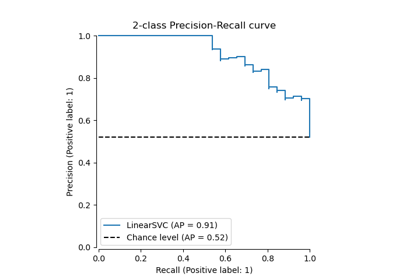# sklearn.metrics.plot_precision_recall_curve¶

sklearn.metrics.plot_precision_recall_curve(estimator, X, y, *, sample_weight=None, response_method='auto', name=None, ax=None, pos_label=None, **kwargs)[source]

Plot Precision Recall Curve for binary classifiers.

Extra keyword arguments will be passed to matplotlib’s plot.

Read more in the User Guide.

Parameters
estimatorestimator instance

Fitted classifier or a fitted Pipeline in which the last estimator is a classifier.

X{array-like, sparse matrix} of shape (n_samples, n_features)

Input values.

yarray-like of shape (n_samples,)

Binary target values.

sample_weightarray-like of shape (n_samples,), default=None

Sample weights.

response_method{‘predict_proba’, ‘decision_function’, ‘auto’}, default=’auto’

Specifies whether to use predict_proba or decision_function as the target response. If set to ‘auto’, predict_proba is tried first and if it does not exist decision_function is tried next.

namestr, default=None

Name for labeling curve. If None, the name of the estimator is used.

axmatplotlib axes, default=None

Axes object to plot on. If None, a new figure and axes is created.

pos_labelstr or int, default=None

The class considered as the positive class when computing the precision and recall metrics. By default, estimators.classes_ is considered as the positive class.

New in version 0.24.

**kwargsdict

Keyword arguments to be passed to matplotlib’s plot.

Returns
displayPrecisionRecallDisplay

Object that stores computed values.

precision_recall_curve
## Examples using sklearn.metrics.plot_precision_recall_curve¶